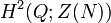# Extensions for trivial outer action of Z2 on SL(2,3)

This article describes all the group extensions corresponding to a particular outer action with normal subgroup special linear group:SL(2,3) and quotient group cyclic group:Z2.

We consider here the group extensions where the base normal subgroup$N$ is special linear group:SL(2,3) (order 24), the quotient group$Q$ is cyclic group:Z2 (order 2), and the induced outer action of the quotient group on the normal subgroup is trivial.

## Description in terms of cohomology groups

We have the induced outer action which is trivial:$Q \to \operatorname{Out}(N)$

Composing with the natural mapping$\operatorname{Out}(N) \to \operatorname{Aut}(Z(N))$, we get a trivial map:$Q \to \operatorname{Aut}(Z(N))$

Thus, the extensions for the trivial outer action of$Q$ on$N$ correspond to the elements of the second cohomology group for trivial group action:$\! H^2(Q;Z(N))$

The correspondence is as follows: an element of$H^2(Q;Z(N))$ gives an extension with base$Z(N)$ and quotient$Q$. We take the central product of this extension group with$N$, identifying the common$Z(N)$.

See second cohomology group for trivial group action of Z2 on Z2, which is isomorphic to cyclic group:Z2.

## Extensions

Cohomology class type Number of cohomology classes Corresponding group extension for$Q$ on$Z(N)$ Second part of GAP ID (order is 4) Corresponding group extension for$Q$ on$N$ (obtained by taking the central product with$N$ of the extension for$Q$ on$Z(N)$) Second part of GAP ID (order is 48) Is the extension a semidirect product of$N$ by$Q$? Is the base characteristic in the whole group? Derived length of whole group
trivial 1 Klein four-group 2 direct product of SL(2,3) and Z2 32 Yes Yes 3
nontrivial 1 cyclic group:Z4 1 central product of SL(2,3) and Z4 13 Yes Yes 3